# Hysteresis

## An R package for fitting rate-dependent hysteretic loops

Hysteresis loops occur when an output variable can have multiple possible values at one input value depending on the history of the system and the direction of change in the input. This package contains functions to simulate, fit, and obtain parameter values along with their standard errors (Yang and Parkhurst) from hysteresis loops of the form $x_{t}=b_{x}cos(2t\pi/T+\phi)+c_{x}+e_{x,t}$ $y_{t}=b_{y}*cos(2t\pi/T+\phi)^{n}+R*sin(2t\pi/T+\phi)^{m}+c_{y}+e_{y,t}$

where e is a random error term. These generalized transcendental equations (Lapshin) form a hysteresis loop for a given frequency or period and set of time points t=1,2…n.

The plot below uses the function mloop which simulates hysteresis loops to show the effects of choosing various odd values for n and m.

library(knitr)
library(hysteresis)
par(mfrow = c(3, 3), mai = c(0, 0.2, 0.2, 0), ann = FALSE, xaxt = "n", yaxt = "n",
oma = c(0, 0, 3, 0))

for (i in c(1, 3, 15)) {
for (j in c(1, 3, 15)) {
obj <- mloop(m = i, n = j, n.points = 100, period = 99)
plot(floop(obj$x, obj$y, m = i, n = j, period = 99), xlim = c(-0.8,
0.8), ylim = c(-0.8, 0.8))
if (i == 1)
title(paste("n=", j, sep = ""))
if (j == 1)
title(ylab = paste("m=", i, sep = ""), line = 0, cex.sub = 2)
}
}
title("Hysteresis Loops for Odd Values of m and n", outer = TRUE)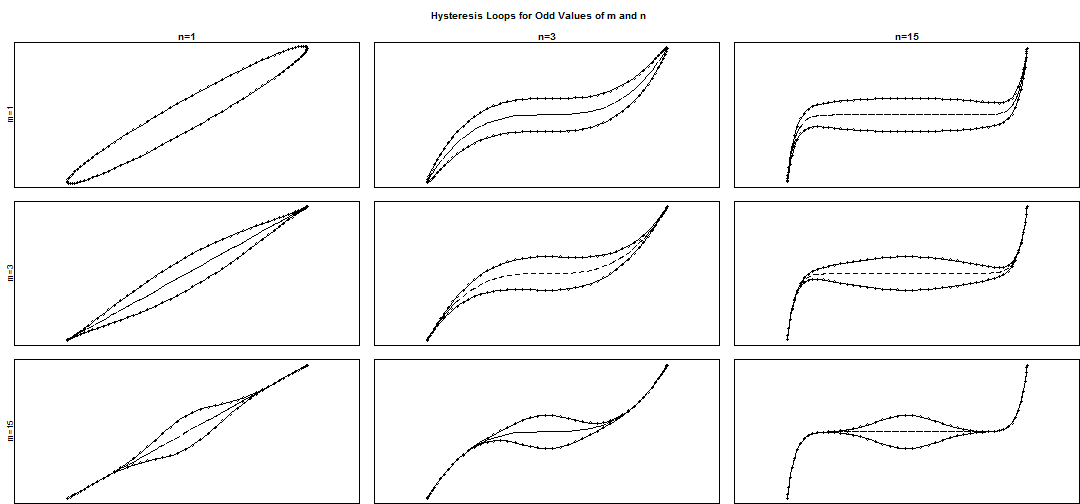It is also possible to use even values for n.


par(mfrow = c(3, 3), mai = c(0, 0.2, 0.2, 0), ann = FALSE, xaxt = "n", yaxt = "n",
oma = c(0, 0, 3, 0))

for (i in c(1, 3, 15)) {
for (j in c(2, 4, 16)) {
obj <- mloop(m = i, n = j, n.points = 100, period = 99)
plot(floop(obj$x, obj$y, m = i, n = j, period = 99), xlim = c(-0.8,
0.8), ylim = c(-0.8, 0.8))
if (i == 1)
title(paste("n=", j, sep = ""))
if (j == 2)
title(ylab = paste("m=", i, sep = ""), line = 0, cex.sub = 2)
}
}
title("Hysteresis Loops for Odd Values of m and Even Values of n", outer = TRUE)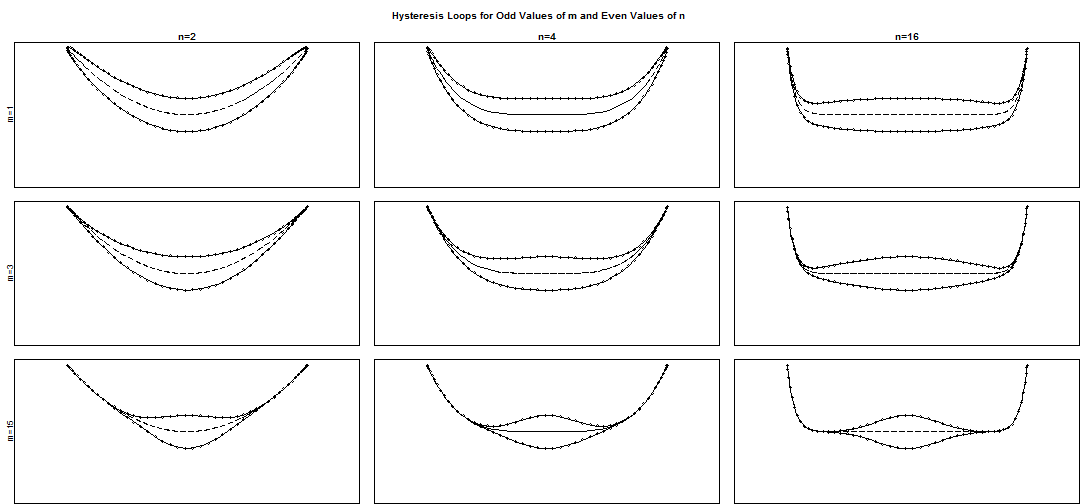A special case is when n=1 and m=1, this makes the hysteresis loop an ellipse. The centroid of the hysteresis loop is given by cx and cy as shown in the plot below of ellipses.

obj <- mloop(cx = 0, cy = 0, n.points = 100, period = 99)
obj2 <- mloop(cx = 1.5, cy = 0, n.points = 100, period = 99)
obj3 <- mloop(cx = 0, cy = 1.5, n.points = 100, period = 99)
plot(obj$x, obj$y, type = "l", xlim = c(-2, 3), ylim = c(-2, 3), xlab = "x",
ylab = "y", col = "#6600CC", main = "Centroid Given by cx and cy")
points(0, 0, pch = 19, col = "#6600CC")
text(x = 0, y = 0.15, "(cx=0,cy=0)", col = "#6600CC")
lines(obj2$x, obj2$y, col = "#00FF66")
points(1.5, 0, pch = 19, col = "#00FF66")
text(x = 1.5, y = 0.15, "(cx=1.5,cy=0)", col = "#00FF66")
lines(obj3$x, obj3$y, col = "#FF6600")
points(0, 1.5, pch = 19, col = "#FF6600")
text(x = 0, y = 1.65, "(cx=0,cy=1.5)", col = "#FF6600")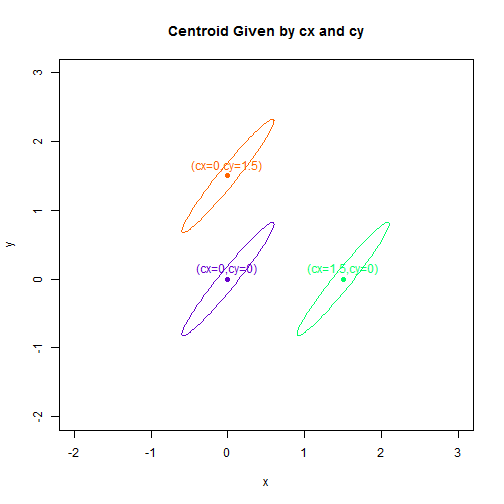The saturation points describe where the direction of the input changes sign. The distances from the central point to the saturation points are given by b.x and b.y (saturation points at ($$c_{x} \pm b_{x},c_{y} \pm b_{y}$$))

for (i in c(1, 2, 4)) {
obj <- mloop(b.x = i, n.points = 100, period = 99)
plot(obj$x, obj$y, xlim = c(-5, 10), ylim = c(-1.4, 1.4), type = "l", main = paste("b.x=",
i, sep = ""), xlab = "x", ylab = "y")
points(i, 0.8, pch = 19)
legend(i, 1, legend = c("Saturation Point", "x=cx+b.x", "y=cy+b.y"), bty = "n")
}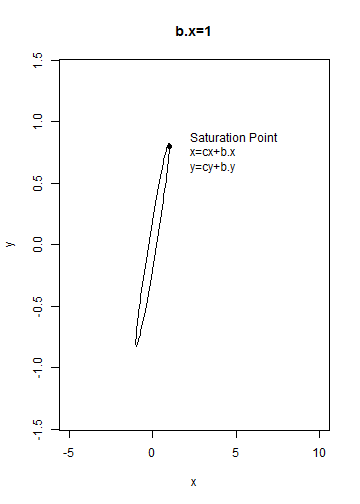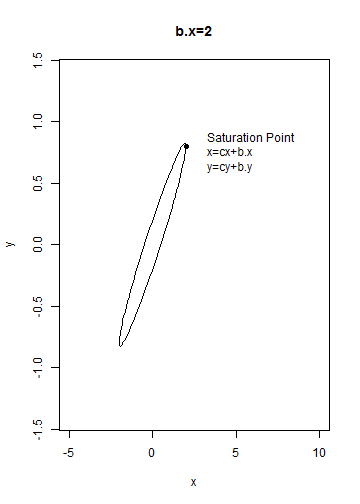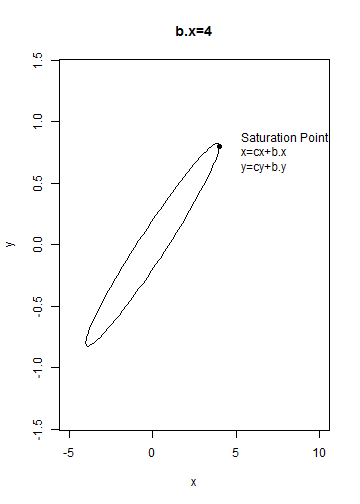for (i in c(0.8, 2, 4)) {
obj <- mloop(b.y = i, n.points = 100, period = 99)
plot(obj$x, obj$y, xlim = c(-1, 2), ylim = c(-5, 5), type = "l", main = paste("b.y=",
i, sep = ""), xlab = "x", ylab = "y")
points(0.6, i, pch = 19)
legend(0.6, i, legend = c("Saturation Point", "x=cx+b.x", "y=cy+b.y"), bty = "n")
}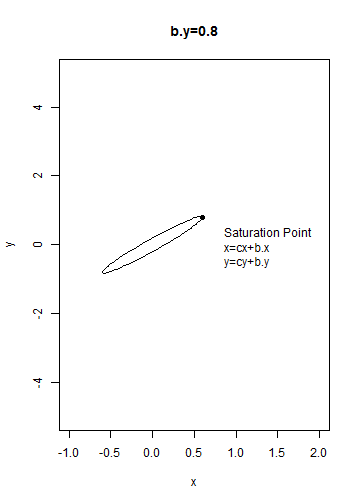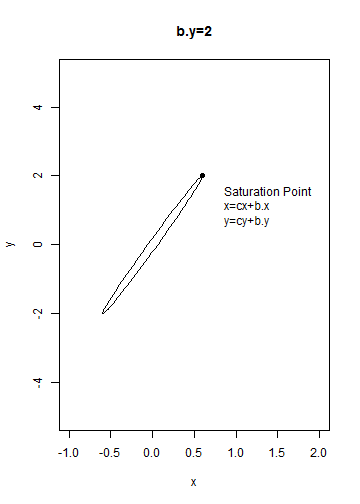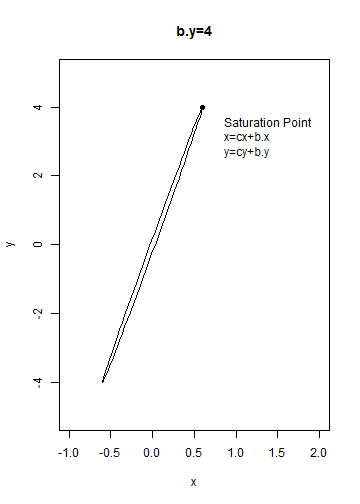Retention, or the output split point R, is the vertical distance from center to upper loop trajectory.

for (i in c(1, 2, 4)) {
obj <- mloop(retention = i, n.points = 100, period = 99)
plot(obj$x, obj$y, xlim = c(-1, 1), ylim = c(-5, 5), type = "l", main = paste("retention=",
i, sep = ""), xlab = "x", ylab = "y")
segments(0, 0, 0, i)
text(0.3, 0.5, "Retention")
}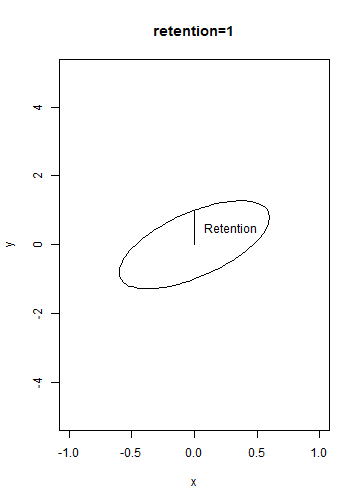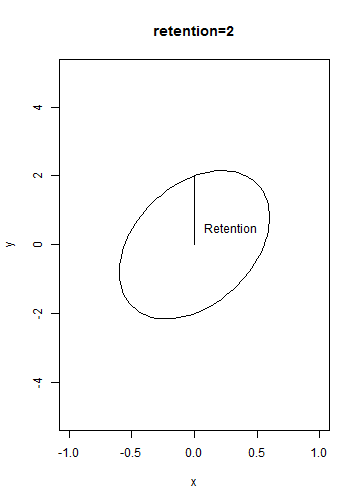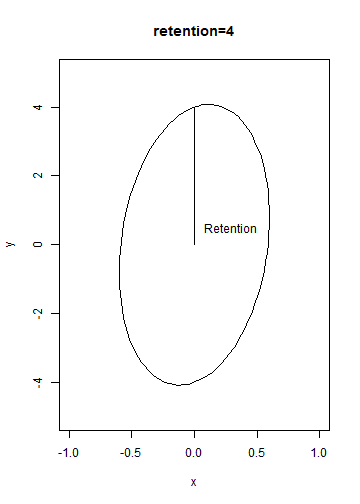Finally the phase.angle, $$\phi$$, changes the location of points along the loop, but does not change the form of the loop itself. When phase.angle is zero, the loop starting point is also the saturation point.

obj <- mloop(retention = 0.5, n.points = 100, period = 99)
plot(obj$x, obj$y, type = "l", xlab = "x", ylab = "y", main = "Starting Points for Different Values of phase.angle",
xlim = c(-0.6, 0.8))
for (i in c(0, 90, 180, 260)) {
obj2 <- mloop(phase.angle = i, retention = 0.5, n.points = 1, period = 99)
points(obj2$x, obj2$y, pch = 19, col = "gold", cex = 2)
points(obj2$x, obj2$y, col = "gold", cex = 4)
text(obj2$x + 0.08, obj2$y, round(i, 2))
}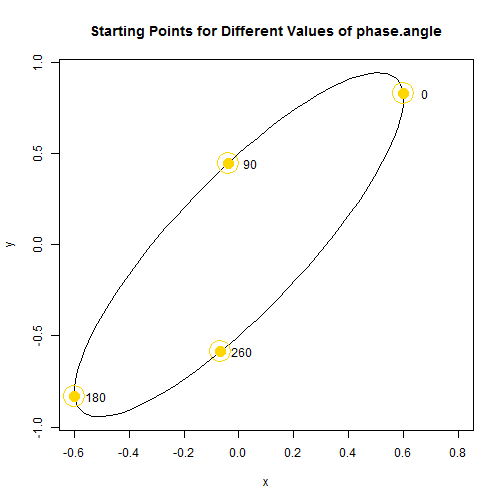## Fitting Ellipses

### The Process

Hysteresis contains one method for fitting hysteresis loops given any n and m in the function floop. In the special case of an ellipse where n=1 and m=1, four methods are available in the function fel. The two-step simple harmonic regression (harmonic2) method, the default, generally produces estimates that are less biased and have lower variances than those produced by the other methods. Since the focus is on rate-dependent hysteresis, knowledge of time for the observations is required (or if unknown, times may be assumed to be equally spaced). On the other hand, if the objective is solely to fit an ellipse, observation times are not needed for the other three methods.

set.seed(24)
ellipse1 <- mel(method = 2, retention = 0.4, b.x = 0.6, b.y = 0.8, cx = 0, cy = 0,
sd.x = 0.1, sd.y = 0.1, phase.angle = 0, period = 24, n.points = 24)
# The function **mel** can be used as an alternative to **mloop** for
# simulating ellipses, and it is useful because it offers four different
# ellipse parameterizations.
model <- fel(ellipse1$x, ellipse1$y, method = "harmonic2", period = 24, times = "equal")
# period=24 and times='equal' are used to say that 24 equally spaced points
# make up an ellipse.
model

## Call:
## fel(x = ellipse1$x, y = ellipse1$y, method = "harmonic2", period = 24,
##     times = "equal")
##
## Delta Method Standard Errors and 95% C.I.'s:
##             Estimates    S.E.      low     high
## b.x          0.611746 0.02348  0.56242  0.66108
## b.y          0.829397 0.03389  0.75819  0.90061
## phase.angle  0.009077 0.03838 -0.07156  0.08971
## cx          -0.013770 0.01660 -0.04865  0.02111
## cy          -0.027886 0.02397 -0.07824  0.02247
## retention    0.423527 0.03389  0.35232  0.49474
## coercion     0.278211 0.02252  0.23090  0.32553
## area         0.813959 0.07224  0.66218  0.96574
## lag          1.803394 0.13902  1.51132  2.09546
## split.angle 53.588291 0.02678 53.53202 53.64456
## ampx         0.611746 0.02348  0.56242  0.66108
## ampy         0.931276 0.03389  0.86007  1.00248
## rote.deg    57.956870 1.53618 54.72947 61.18427


In addition to the fundamental values of the model, fel also calculates a wide variety of derived parameters. Definitions for these parameters can be found using help(loop.parameters).

model$Estimates  ## b.x b.y phase.angle cx cy ## 0.611746 0.829397 0.009077 -0.013770 -0.027886 ## retention coercion area lag split.angle ## 0.423527 0.278211 0.813959 1.803394 53.588291 ## hysteresis.x hysteresis.y ampx ampy rote.deg ## 0.454782 0.510645 0.611746 0.931276 57.956870 ## semi.major semi.minor focus.x focus.y eccentricity ## 1.088509 0.238024 0.563540 0.900344 0.975799  A wide variety of functions have S3 methods for objects of class ellipsefit produced by fel. The most important of these is summary.ellipsefit which can be used to bootstrap and summarize models produced by fel. summary(model, N = 10000, studentize = TRUE)  ## Summary Call: ## summary.ellipsefit(object = model, N = 10000, studentize = TRUE) ## Call for Original Fit: ## fel(x = ellipse1$x, y = ellipse1$y, method = "harmonic2", period = 24, ## times = "equal") ## Ellipse Fitting Method: ##  "harmonic2" ##  "Two step simple harmonic least squares" ## ## Bootstrapped Estimates: ## Boot.Estimate Bias Std.Error B.q0.025 B.q0.975 ## b.x 0.611257 4.890e-04 0.025729 0.56050 0.66074 ## b.y 0.829578 -1.814e-04 0.040574 0.74947 0.90953 ## phase.angle 0.009612 -5.353e-04 0.041290 -0.07215 0.09057 ## cx -0.013768 -2.264e-06 0.017885 -0.04918 0.02197 ## cy -0.027827 -5.919e-05 0.025935 -0.07389 0.02745 ## retention 0.423893 -3.658e-04 0.050428 0.32541 0.52296 ## coercion 0.278577 -3.659e-04 0.033464 0.21345 0.34457 ## area 0.813970 -1.098e-05 0.103135 0.61505 1.01538 ## lag 1.804363 -9.691e-04 0.219718 1.37714 2.24002 ## split.angle 53.641591 -5.330e-02 1.768993 50.08617 57.09629 ## hysteresis.x 0.455761 -9.793e-04 0.051108 0.35426 0.55493 ## hysteresis.y 0.508806 1.839e-03 0.073168 0.37276 0.66000 ## ampx 0.611257 4.890e-04 0.025729 0.56050 0.66074 ## ampy 0.930063 1.213e-03 0.036292 0.85788 1.00195 ## rote.deg 57.940378 1.649e-02 1.656099 54.71641 61.18337 ## rote.rad 1.011250 2.878e-04 0.028904 0.95498 1.06785 ## semi.major 1.087134 1.375e-03 0.033710 1.02035 1.15463 ## semi.minor 0.238329 -3.051e-04 0.029181 0.18182 0.29592 ## focus.x 0.563723 -1.826e-04 0.027569 0.50798 0.61641 ## focus.y 0.899537 8.067e-04 0.037667 0.82449 0.97335 ## eccentricity 0.976133 -3.341e-04 0.006264 0.96234 0.98691  Another important S3 method is for the function plot.  plot(model, main = "2-step Simple Harmonic Regression Ellipse Example")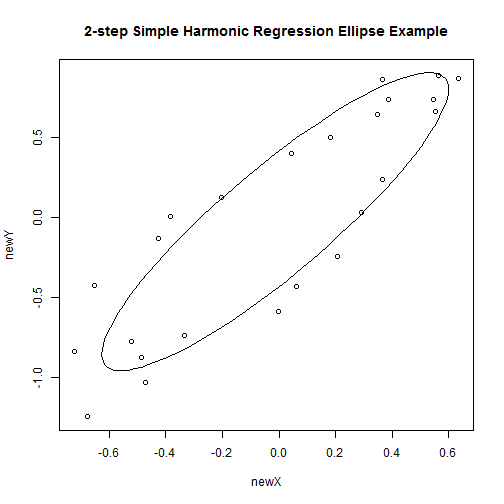In addition, S3 methods exist for fitted, print, and residuals. ### Comparison of Ellipse Estimation Methods The two most useful ellipse estimation methods implemented by fel are the 'harmonic2' and 'direct' methods. The 'direct' method (Flusser and Halir) fits an ellipse without requiring time information and is more stable than the other two methods in fel, 'lm' and 'nls', which are based on linear least squares and ellipse-specific nonlinear regression respectively. The 'direct' method does not yet have delta method standard errors available. modeldirect <- fel(ellipse1$x, ellipse1$y, method = "direct", period = 24, times = "equal") summodel <- summary(modeldirect, N = 10000, studentize = TRUE) summodel  ## Summary Call: ## summary.ellipsefit(object = modeldirect, N = 10000, studentize = TRUE) ## Call for Original Fit: ## fel(x = ellipse1$x, y = ellipse1$y, method = "direct", period = 24, ## times = "equal") ## Ellipse Fitting Method: ##  "direct" ##  "Direct specific least squares" ## ## Bootstrapped Estimates: ## Boot.Estimate Bias Std.Error B.q0.025 B.q0.975 ## b.x 0.6453 -0.013049 0.027419 0.5938 0.70141 ## b.y 0.8142 -0.044763 0.046575 0.7235 0.90573 ## cx -0.1001 0.008285 0.026764 -0.1507 -0.04593 ## cy -0.1531 0.012602 0.032522 -0.2216 -0.09368 ## retention 0.4441 0.037435 0.034189 0.3797 0.51347 ## coercion 0.3108 0.024614 0.025031 0.2639 0.36206 ## area 0.9042 0.052129 0.066896 0.7771 1.04005 ## lag 1.8946 0.241225 0.199886 1.5227 2.30861 ## split.angle 51.7340 -1.142141 1.848919 47.8950 55.19121 ## hysteresis.x 0.4788 0.051640 0.042314 0.3979 0.56411 ## hysteresis.y 0.5311 0.094669 0.080586 0.3898 0.70684 ## ampx 0.6453 -0.013049 0.027419 0.5938 0.70141 ## ampy 0.9228 -0.015138 0.034047 0.8614 0.99595 ## rote.deg 56.1949 0.583768 1.704864 52.9543 59.59864 ## rote.rad 0.9808 0.010189 0.029755 0.9242 1.04019 ## semi.major 1.0962 -0.027294 0.037634 1.0285 1.17661 ## semi.minor 0.2611 0.023675 0.021696 0.2205 0.30587 ## focus.x 0.5928 -0.028371 0.033590 0.5290 0.66022 ## focus.y 0.8867 -0.024809 0.039220 0.8135 0.96847 ## eccentricity 0.9732 -0.009324 0.008429 0.9543 0.98744  plot(modeldirect, main = "Direct Ellipse Example")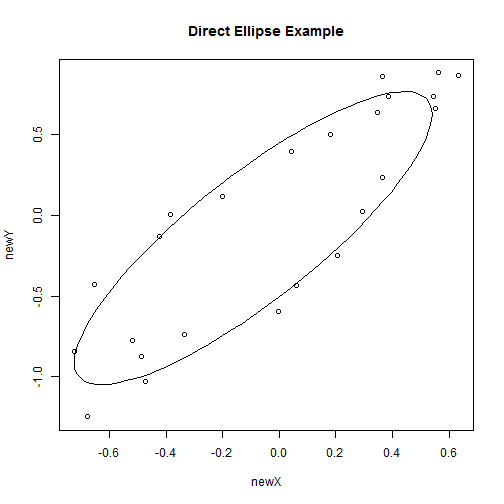The 'direct' method uses different fundamental parameters than the 'harmonic2' method. However summary results for b.x, b.y, and retention are still available from the matrix of values produced by summary.ellipsefit. summodel$values

##              Orig.Estimate B.q0.025 B.q0.25  B.q0.5  B.q0.75 B.q0.975
## b.x                0.63223   0.5938  0.6263  0.6445  0.66323  0.70141
## b.y                0.76946   0.7235  0.7824  0.8135  0.84463  0.90573
## cx                -0.09181  -0.1507 -0.1183 -0.1007 -0.08255 -0.04593
## cy                -0.14053  -0.2216 -0.1733 -0.1518 -0.13100 -0.09368
## retention          0.48151   0.3797  0.4206  0.4428  0.46650  0.51347
## coercion           0.33538   0.2639  0.2935  0.3095  0.32729  0.36206
## area               0.95637   0.7771  0.8575  0.9036  0.94886  1.04005
## lag                2.13580   1.5227  1.7580  1.8883  2.02282  2.30861
## split.angle       50.59186  47.8950 50.5386 51.8236 52.99894 55.19121
## hysteresis.x       0.53047   0.3979  0.4502  0.4783  0.50657  0.56411
## hysteresis.y       0.62577   0.3898  0.4752  0.5256  0.58018  0.70684
## ampx               0.63223   0.5938  0.6263  0.6445  0.66323  0.70141
## ampy               0.90770   0.8614  0.8995  0.9212  0.94395  0.99595
## rote.deg          56.77868  52.9543 55.0285 56.1821 57.33336 59.59864
## rote.rad           0.99097   0.9242  0.9604  0.9806  1.00066  1.04019
## semi.major         1.06889   1.0285  1.0702  1.0940  1.11988  1.17661
## semi.minor         0.28480   0.2205  0.2460  0.2601  0.27563  0.30587
## focus.x            0.56445   0.5290  0.5700  0.5924  0.61498  0.66022
## focus.y            0.86186   0.8135  0.8602  0.8851  0.91171  0.96847
## eccentricity       0.96385   0.9543  0.9680  0.9740  0.97916  0.98744
##              Std.Error Boot.Mean      Bias Boot.Estimate
## b.x           0.027419   0.61918 -0.013049        0.6453
## b.y           0.046575   0.72470 -0.044763        0.8142
## cx            0.026764  -0.08353  0.008285       -0.1001
## cy            0.032522  -0.12793  0.012602       -0.1531
## retention     0.034189   0.51894  0.037435        0.4441
## coercion      0.025031   0.35999  0.024614        0.3108
## area          0.066896   1.00850  0.052129        0.9042
## lag           0.199886   2.37703  0.241225        1.8946
## split.angle   1.848919  49.44971 -1.142141       51.7340
## hysteresis.x  0.042314   0.58211  0.051640        0.4788
## hysteresis.y  0.080586   0.72044  0.094669        0.5311
## ampx          0.027419   0.61918 -0.013049        0.6453
## ampy          0.034047   0.89256 -0.015138        0.9228
## rote.deg      1.704864  57.36245  0.583768       56.1949
## rote.rad      0.029755   1.00116  0.010189        0.9808
## semi.major    0.037634   1.04159 -0.027294        1.0962
## semi.minor    0.021696   0.30848  0.023675        0.2611
## focus.x       0.033590   0.53608 -0.028371        0.5928
## focus.y       0.039220   0.83706 -0.024809        0.8867
## eccentricity  0.008429   0.95453 -0.009324        0.9732


The four plots below illustrate a comparison of the four methods for fitting an ellipse to simulated data. The data points are based on the simulated red ellipse; the fitted ellipse is in black.

set.seed(13)
par(mfrow = c(2, 2))
halfellipse <- mel(method = 2, cx = 20, cy = 25, retention = 1.2, b.x = 14,
b.y = 0.8, sd.x = 1, sd.y = 0.2, period = 24, n.points = 16, phase.angle = pi/2)
halftrueellipse <- mel(method = 2, cx = 20, cy = 25, retention = 1.2, b.x = 14,
b.y = 0.8, phase.angle = 0, period = 99, n.points = 100)
harmodel <- fel(halfellipse$x, halfellipse$y, method = "harmonic2", period = 24,
times = "equal")
dirmodel <- fel(halfellipse$x, halfellipse$y, method = "direct", period = 24,
times = "equal")
lmmodel <- fel(halfellipse$x, halfellipse$y, method = "lm", period = 24, times = "equal")
nlsmodel <- fel(halfellipse$x, halfellipse$y, method = "nls", period = 24, times = "equal",
control = c(n.iter = 500))
plot(harmodel, main = "Harmonic2 Model", xlim = c(5, 34), ylim = c(23.4, 26.9))
lines(halftrueellipse$x, halftrueellipse$y, col = "red")
plot(dirmodel, main = "Direct Model", xlim = c(5, 34), ylim = c(23.4, 26.9))
lines(halftrueellipse$x, halftrueellipse$y, col = "red")
plot(lmmodel, main = "Linear Model", xlim = c(5, 34), ylim = c(23.4, 26.9))
lines(halftrueellipse$x, halftrueellipse$y, col = "red")
plot(nlsmodel, main = "Non-Linear Model", xlim = c(5, 34), ylim = c(23.4, 26.9))
lines(halftrueellipse$x, halftrueellipse$y, col = "red")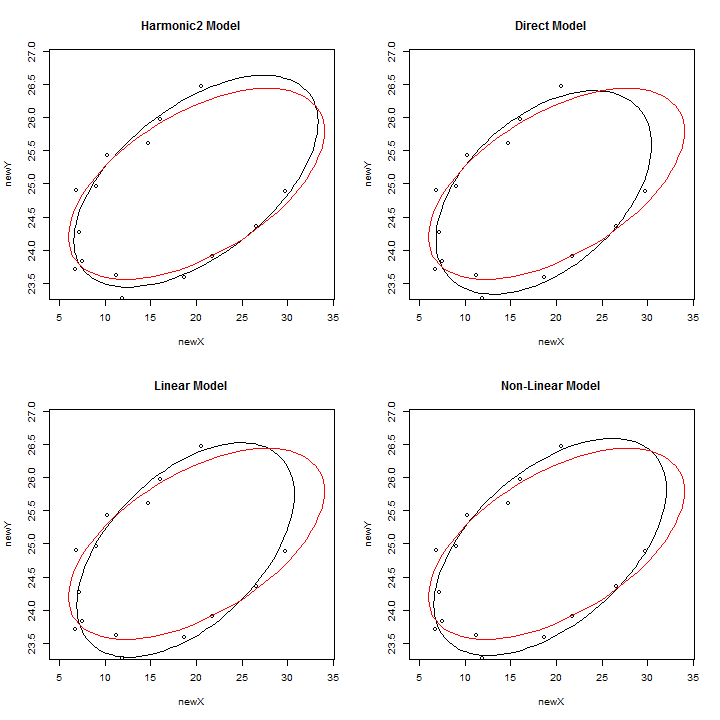## Geometric Method

The geometric ellipse method used here is based on the work of Gander, Golub and Strebel, and the code used is an R translation of the Matlab code they provided. This method directly minimizes the Euclidean distances from the ellipse through Gauss-Newton minimization. Standard errors are obtainable through either the Delta Method and bootstrapping, however the use of bootstrapping through summary.ellipsefit is discouraged on these ellipses as the geometric method is extremely computationally expensive. The “geometric” method works best when sd.x=sd.y, and it is important to check for false convergence. One way to check for false convergence is to examine the plot after fitting the data.

set.seed(101)
ellip <- mel(rote.deg = 45, semi.major = 5, semi.minor = 3, n.points = 13, sd.x = 0.4,
sd.y = 0.4)
true.ellip <- mel(rote.deg = 45, semi.major = 5, semi.minor = 3, n.points = 100,
period = 100)
ellip.geometric <- fel(ellip$x, ellip$y, method = "geometric")
## write.table(summodels$Boot.Estimates,'file_name.txt')  ## Fitting Hysteresis Loops The function floop can be used to fit hysteresis loops with specific values of n and m as arguments to floop. Below is an example of a hysteresis loop with n=5, m=3. loop <- mloop(n = 5, m = 3, sd.x = 0.02, sd.y = 0.02) fitloop <- floop(loop$x, loop$y, n = 5, m = 3, period = 24, times = "equal")  ## Warning: hysteresis.x and coercion only available if m=n  fitloop$Estimates

##                n                m              b.x              b.y
##         5.000000         3.000000         0.609974         0.797298
##      phase.angle               cx               cy        retention
##        -0.012900        -0.003235        -0.001433         0.198210
##         coercion             area              lag beta.split.angle
##               NA         0.284870         0.930721         0.000000
##     hysteresis.x     hysteresis.y
##               NA         0.248602

plot(fitloop, main = "Fitted Hysteresis Loop")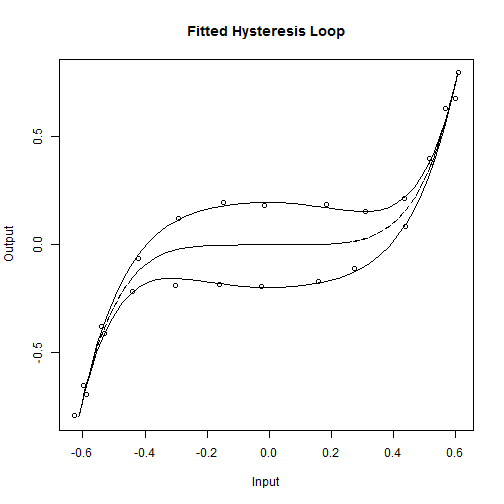summary(fitloop)

## Summary Call:
## summary.fittedloop(object = fitloop)
## Call for Original Fit:
## floop(x = loop$x, y = loop$y, n = 5, m = 3, times = "equal",
##     period = 24)
##
## Bootstrapped Estimates:
##                  Boot.Estimate       Bias Std.Error  B.q0.025  B.q0.975
## n                     5.000000  0.000e+00  0.000000  5.000000  5.000000
## m                     3.000000  0.000e+00  0.000000  3.000000  3.000000
## b.x                   0.610017 -4.301e-05  0.003549  0.603288  0.617060
## b.y                   0.797376 -7.876e-05  0.007621  0.782977  0.812776
## phase.angle          -0.025725  1.283e-02  0.006019 -0.037573 -0.013901
## cx                   -0.003184 -5.078e-05  0.002644 -0.008492  0.001719
## cy                   -0.001226 -2.073e-04  0.003730 -0.008998  0.005879
## retention             0.198355 -1.456e-04  0.006739  0.184992  0.211902
## coercion                    NA         NA        NA        NA        NA
## area                  0.285099 -2.289e-04  0.009838  0.265076  0.305437
## lag                   0.931271 -5.498e-04  0.031658  0.867552  0.994353
## beta.split.angle      0.000000  0.000e+00  0.000000  0.000000  0.000000
## hysteresis.x                NA         NA        NA        NA        NA
## hysteresis.y          0.248736 -1.347e-04  0.008801  0.231079  0.266327


## Acknowledgments

NIFA MRF 25-008/W-2173 Impacts of Stress Factors on Performance, Health, and Well Being of Farm Animals